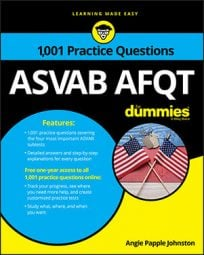##### ASVAB AFQTThe Arithmetic Reasoning subtest on the ASVAB contains only word problems. While most of them are straightforward, they may also include extra information that distracts you from pulling out the data you need.

Don't let the extra information in these problems throw you off. Try the following practice questions to super-size your powers of deduction—or rather, reduction.

## Practice questions

1. Charra and Glenn have teamed up to create a website for a client. Charra bills \$110 per hour, and Glenn bills \$95 per hour. How long would it take Charra to build the website alone if the total cost to the client were \$4,750?

A. 45.43 hours B. 38.7 hours C. 42.12 hours D. 43.18 hours

2. Specialists Vasquez and Wolley were told to sweep the company's motor pool. Vasquez started at 10 a.m. and swept a 50-foot by 50-foot portion of the motor pool, and Wolley began at 10:45 a.m. and swept the remainder of the motor pool, which was just as wide but 15 feet shorter than what Vasquez swept. What is the motor pool's total area?

A. 4,250 square feet B. 4,475 square feet C. 5,000 square feet D. 3,850 square feet

1. The correct answer is Choice (D).

Don't let extra information confuse you on the ASVAB. You don't need to know how much Glenn charges at all; you only need to know how much Charra charges, which is \$110 per hour.

The problem tells you how much the project costs (\$4,750), but it doesn't tell you how long it takes to complete at \$110 per hour. Let x represent the amount of time it takes Charra to complete \$4,750 worth of work on a website, and create your equation with what you know:

It takes Charra about 43.18 hours to complete a website that costs \$4,750.

If you needed to know how long it would take Glenn, you could use the same formula with his hourly rate.

2. The correct answer is Choice (A).

What time each soldier started sweeping doesn't matter, because the problem only asks you to identify the motor pool's area.

You can draw a sketch of the motor pool so you can visualize the part that each specialist swept:

You know that Wolley's portion of the motor pool was 50 feet by 35 feet (the problem tells you that his portion was 15 feet shorter than Vasquez's area).

Figure out the area each soldier swept and add them together: